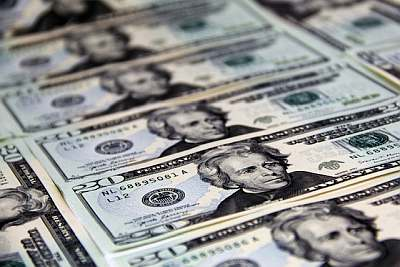# Times Interest Earned Ratio

Instructions: Use this Times Interest Earned Ratio to compute the Times Interest Earned Ratio $$(TIER)$$ with this calculator. You need to provide the Earnings Before Interest and Taxes $$(EBIT)$$ and the interest paid in the form below:Earnings Before Interest and Taxes $$(EBIT)$$ =Intertest =

## Times Interest Earned Ratio Calculator

### Time Interest Earned Ratio Formula

The Times Interest Earned Ratio corresponds to the ratio between the EBIT and the interest. This ratio is a measure of long term solvency, and it indicates the number many times earnings can pay for the interest owed:

$TIER = \displaystyle \frac{EBIT}{\text{Interest}}$### Example: Calculation of Times Interest Earned Ratio

Question: Consider a firm that has earnings before interest and taxes of $43,000, and it has an interest due of$3,400. Compute the corresponding Times Interest Earned Ratio.

Solution:

This is the information we have been provided with:

• The earnings before interest and taxes is $$EBIT = 43000$$ and the interest $$Interest = 3400$$.

Hence, the times interest earned ratio $$TIER$$ is computed using the following formula:

$\begin{array}{ccl} TIER & = & \displaystyle \frac{EBIT}{\text{Interest}} \\\\ \\\\ & = & \displaystyle \frac{43000}{3400} \\\\ \\\\ & = & 12.65 \end{array}$

Therefore, the times interest earned ratio, for the given earnings before interest and taxes of $$EBIT = 43000$$, and interest of $$3400$$, is equal to $$TIER = 12.65$$. This means that firm's earnings before interest and taxes are $$12.65$$ times its due interest.

### Calculation of Commonly Used Financial Ratios

The Times Interest Earned Ratio is a commonly used financial ratio in the study of corporate finance. You can find other financial ratio calculator in our site, such as our current ratio , quick ratio , inventory turnover calculator.

A tightly related profit ratio is the Cash Coverage Ratio , which usually reported along with the Times Interest Earned Ratio.